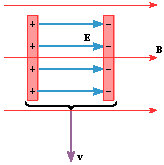# Magnetic Fields & Forces

• jason.maran

## Homework Statement

The drawing shows a parallel plate capacitor that is moving with a speed of 31 m/s through a 4.0 T magnetic field. The velocity v is perpendicular to the magnetic field. The electric field within the capacitor has a value of 170 N/C and each plate has an area of 7.5 multiplied by 10 x 10^-4 m^2. What is the magnetic force (magnitude and direction) exerted on the positive plate of the capacitor?## Homework Equations

F = Bqvsin(theta) --- sin() isn't important in this case because it's perpendicular

Then there's also E = F/q and C = KEoA/d, but I can't for the life of me figure out how to use it in this case.

## The Attempt at a Solution

Well, I've established that I'm going to need to find the charge somehow from the electric field and the area of the plates, since all the other info for F = Bvq is given. I'm at a loss to figure out how to get the charge though... thanks in advance for any help/advice.

#### Attachments

You're completely correct. As it turns out, if you draw a Guassian cylinder or cube that extends halfway into one of the (conducting) capacitor plates and apply Gauss's Law, you'll find that the electric field from one plate is always $$\sigma/2\epsilon_0$$, where $$\sigma$$ is the charge density of the plate (charge over area). If you add to this value the field from the other plate, you'll find that inside a parallel plate capacitor, the electric field always has a value of $$\sigma/\epsilon_0$$. So given your value for the electric field and the areas of the plates, you can now calculate the charge on the positive plate.

Thanks for your help, swuster. I'm still having a bit of trouble though.

I've done the following now:

E = (q/A)/2Eo
170 = (q/7.5e-4)/(2 * 8.85e-12)
q = 2.25e-12 C

Then I substitute in and solve for F:
F = qvB = (2.25e-12)(31)(4) = 2.79e-10 N, which is wrong, unfortunately.

I feel like I missed a step when following your instructions, and I didn't quite follow this bit:
If you add to this value the field from the other plate, you'll find that inside a parallel plate capacitor, the electric field always has a value of LaTeX Code: \\sigma/\\epsilon_0 .
Thanks again for the help, I appreciate it greatly! :)

E = (q/A)/2Eo

Should be:

E = (q/A)/Eo

^_^

Ah, OK, thanks a bunch! Worked like a charm after that!

Yep - between the capacitor plates, the electric field adds!